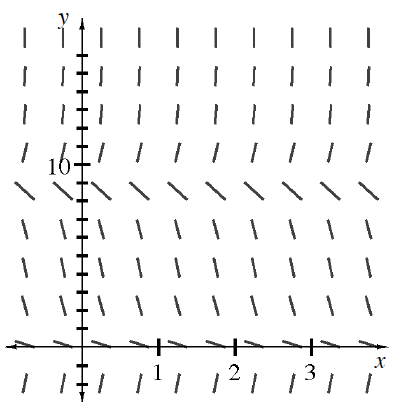### Home > APCALC > Chapter 10 > Lesson 10.1.8 > Problem10-102

10-102.

Examine the slope field shown at right. Imagine drawing some solutions, beginning with a variety of $y$-intercepts above the $x$-axis. What will be $\lim\limits _ { x \rightarrow - \infty } y ( x )$ for each of the solutions?

Where are the possible horizontal asymptotes for the solution curve?